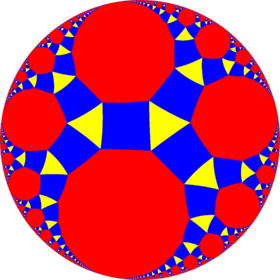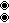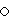# Rhombitriapeirogonal tiling

Rhombitriapeirogonal tilingPoincaré disk model of the hyperbolic plane
TypeHyperbolic uniform tiling
Vertex configuration3.4..4
Schläfli symbolrr{,3} ors2{3,}
Wythoff symbol3 | 2
Coxeter diagramorSymmetry group[,3], (*32)
[,3+], (3*)
DualDeltoidal triapeirogonal tiling
PropertiesVertex-transitive

In geometry, the rhombtriapeirogonal tiling is a uniform tiling of the hyperbolic plane with a Schläfli symbol of rr{∞,3}.

## Symmetry

This tiling has [∞,3], (*∞32) symmetry. There is only one uniform coloring.

Similar to the Euclidean rhombitrihexagonal tiling, by edge-coloring there is a half symmetry form (3*∞) orbifold notation. The apeireogons can be considered as truncated, t{∞} with two types of edges. It has Coxeter diagram, Schläfli symbol s2{3,∞}. The squares can be distorted into isosceles trapezoids. In the limit, where the rectangles degenerate into edges, an infinite-order triangular tiling results, constructed as an snub triapeirotrigonal tiling,.

### Symmetry mutations

This hyperbolic tiling is topologically related as a part of sequence of uniform cantellated polyhedra with vertex configurations (3.4.n.4), and [n,3] Coxeter group symmetry.Wikimedia Commons has media related to Uniform tiling 3-4-i-4.